Simple Harmonic Motion | Spring Mass System

Let’s explore different spring mass systems, with mass under linear or rotational motion, and determine angular frequency or time period of simple harmonic motion/oscillations of such a system. More specifically we will look at the following scenarios

Spring Mass System | Linear SHM | Springs in SeriesFor springs in series, tension or restoring force exerted by two springs in series is same, i.e. $F = -k_1 x_1 = -k_2 x_2$ or $x_1 + x_2 = x = -F(\cfrac{1}{k_1}+\cfrac{1}{k_2})$
So, equivalent stiffness, $k_{eq} =-\cfrac{F}{x}= \cfrac{k_1 k_2}{k_1+k_2}$
and, angular frequency, $\omega = \sqrt{\cfrac{k_{eq}}{m}}$
and, time period $T = \cfrac{2 \pi}{\omega}$

Spring Mass System | Linear SHM | Springs in Parallel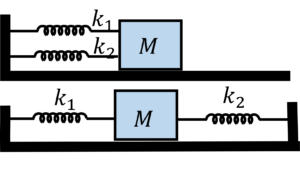For springs in parallel, the amount of extension, $x$, is the same
and equivalent stiffness $k_{eq} = k_1 + k_2$
so, angular frequency, $\omega = \sqrt{\cfrac{k_{eq}}{m}}$

Spring Mass System | Linear SHM | Multiple Springs in 2D ArrangementGiven that the mass is allowed to move along the $y-$axis (dotted line),
For a small displacement, $\delta$, of mass $m$ (from its mean position) along say positive $y$-axis, the restoring force, $F$ will be $=(k_1 + 2 k_2 \cos^2 \theta) \delta$
So, $k_{eq} = k_1 + 2 k_2 \cos^2 \theta$
and angular frequency, $\omega = \sqrt{\cfrac{k_{eq}}{m}}$

Spring Mass System | 2D SHMIf $k_1 = k_2$, then the mass will move along a straight line

If $k_1 = 4k_2$, meaning $\omega_1 = 2 \omega_2$ then the particle will trace a horizontal 8 as shown. Note that as the particle completes 1 oscillation in the horizontal direction, it completes two oscillations in the vertical direction
(and if $4k_1 = k_2$, then the particle will trace the standing or vertical 8)

You can use similar approach to determine the resulting trajectory of the particle for other combinations of $k_1$ and $k_2$.

Spring Mass System | Linear SHM | Pulley - Spring - Mass System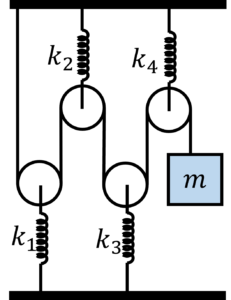Assuming that the pulleys are massless, let’s say that we displace the mass $m$ by a small distance $\delta$ in the downward direction (from its mean or equilibrium position), let’s figure out the increase (or change) in tension.
so, let’s say that the increase in tension is $T$,
then
Increase in stretching of spring $1$, $\delta_1 = \cfrac{2T}{k_1}$
Similarly, increase in stretching of spring $2$ will be $\delta_2 = \cfrac{2T}{k_2}$
$\delta_3 = \cfrac{2T}{k_3}$
$\delta_4 = \cfrac{2T}{k_4}$

And $2 \delta_1 + 2 \delta_2 + 2 \delta_3 + 2 \delta_4 = \delta$

So, $4T (\cfrac{1}{k_1} + \cfrac{1}{k_2} + \cfrac{1}{k_3} + \cfrac{1}{k_4}) = \delta$

Now, $k_{eq} = \cfrac{T}{\delta}$
i.e. $k_{eq} = \cfrac{1}{4(\cfrac{1}{k_1} + \cfrac{1}{k_2} + \cfrac{1}{k_3} + \cfrac{1}{k_4})}$

& angular frequency $\omega = \sqrt{\cfrac{k_{eq}}{m}}$

Spring Mass System | Linear SHM | Constant ForceNote that, in the equilibrium position spring is compressed by $\cfrac{mg \sin \theta}{k}$ where $\theta$ is the angle of incline, assuming the incline surface is frictionless

Now if we displace mass $m$ slightly from its mean or equilibrium position, it will oscillate with angular frequency $\omega = \sqrt{\cfrac{k}{m}}$

Linear SHM | Two particles executing SHM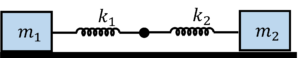If we displace masses $m_1$ and $m_2$ (connected by spring of spring constant $k_{eq}$) towards each other and then release them, they will undergo simple harmonic motion (with their center of mass at rest)

So, let’s write the newton’s law $F = ma = m \cfrac{d^2 x}{dt^2}$ for mass $m_1$, when it is a distance $\delta_1$ from its mean position towards left

Well to determine the restoring spring force $F$, we need to know the total extension of the spring when $m_1$ is at $\delta_1$ to the left of its mean position.

Well recall that $x_{cm} = \cfrac{m_1 x_1 + m_2 x_2}{m_1 + m_2}$

Now if you take origin at center of mass then $m_1 x_1 + m_2 x_2 = 0$ or $m_1 \delta_1 + m_2 \delta_2 = 0$ or $\delta_2 = – \cfrac{m_1}{m_2} \delta_1$ with minus sign indicating that if $\delta_1$ was towards left $\delta_2$ will be towards right

so net extension of the spring $=\delta_1 + \delta_2 = \cfrac{m_1 + m_2}{m_2} \delta_1$

or, $-k_{eq} \cfrac{m_1 + m_2}{m_2} \delta_1$ $=m_1 \cfrac{d^2 \delta_1}{dt^2}$

or $\cfrac{d^2 \delta_1}{dt^2} = – \cfrac{k_{eq}}{\cfrac{m_1 m_2}{m_1+m_2}} \delta_1$

i.e. $\omega^2 = \cfrac{k_{eq}}{\cfrac{m_1 m_2}{m_1+m_2}}$

Angular SHM | Scenario 1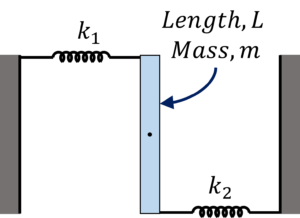If we rotate the rod by a small angle $\theta$ about its center, the springs will be stretched by $\cfrac{L}{2} \theta$

So, the restoring torque about the pivot point will be $\tau = \cfrac{L}{2}$$(k_1 \cfrac{L}{2} \theta +$ $k_2 \cfrac{L}{2} \theta)$ $=\cfrac{L^2}{4} (k_1 + k_2) \theta$

Now, as per newton’s 2nd law $\tau = I \alpha = I \cfrac{d^2 \theta}{dt^2}$

So, $\cfrac{d^2 \theta}{dt^2}=-\cfrac{L^2}{4I} (k_1 + k_2) \theta$, where $I = \cfrac{1}{12} ML^2$ for the rod

So angular frequency, $\omega^2 = \cfrac{L^2}{4I} (k_1 + k_2)$

Note that above is true only for small angular displacements. For larger angular displacements the motion will not be simple harmonic

Angular SHM | Scenario 2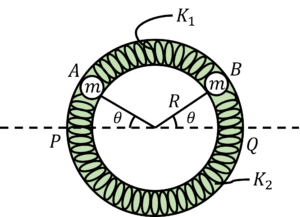Note that assuming that the springs are ideal springs, motion will be simple harmonic ir-respective of how large the angular displacement, $\theta$, is from its mean equilibrium position of $PQ$

Here the restoring force on each mass is $2 k_1 R \theta + 2 k_2 R \theta$ $=2(k_1 + k_2) R \theta$

So, restoring torque on mass $m$ is $2(k_1 + k_2) R^2 \theta$

Now, writing netwon’s 2nd law for mass $m$, $\tau = I \alpha$ $= I \cfrac{d^2 \theta}{dt^2}$ $mR^2 \cfrac{d^2 \theta}{dt^2}$

i.e. $\cfrac{d^2 \theta}{dt^2} = – \cfrac{2(k_1+k_2)}{m} \theta$

or, angular frequency $\omega = \sqrt{\cfrac{2(k_1+k_2)}{m}}$

Simple Harmonic Motion | Linear + Rotational Motion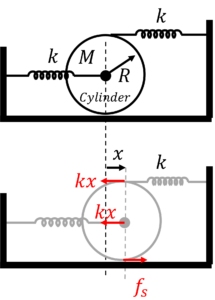Assuming that cylinder is in pure rolling at all times, let’s say that the center of cylinder is displaced by a distance $x$ to the right as shown, then the net restoring force acting on it is

$F_{net} = -(2kx – f_s)$ (assuming that the friction force is acting to the right)

Newton’s 2nd law ($F_{net}=Ma_{cm}$) gives us
$-(2kx – f_s) = M a_{cm}$ $\cdots$(i)

Now torque on cylinder about its center of mass $\tau_{net} =-(kx+f_s)R$

Using newton’s 2nd law $\tau_{net} = I \alpha$ we get
$-(kx+f_s)R = I \alpha$

Now in pure rolling $a_{cm} = \alpha R$
i.e. $-(kx+f_s) = a_{cm} \cfrac{I}{R^2}$ $\cdots$(ii)

Solving for $a_{cm}$ in terms of $x$ we get
$a = \cfrac{d^2x}{dt^2}=$ $-\cfrac{3k}{M + \cfrac{I}{R^2}} x$

or angular frequency $\omega = \sqrt{\cfrac{3k}{M + \cfrac{I}{R^2}}}$